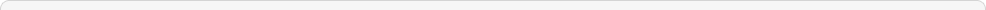Video Tutorials
Getting Started Tutorial and Demo
5 March , 2011
18007 views
Text & ArticlesCreated : Sat , 05 November 2011 21:20   Views : 2410
This is Working Capital Per Share divided by the recent interim period Price Close. Working Capital Per Share is defined as the difference between Current Assets and Current Liabilities for the most recent interim period divided by the Total Common Shares Outstanding at the end of that same period. NOTE: This item is reported only for Industrial and Utility companies.Created : Sat , 05 November 2011 21:20   Views : 2439
This value is the current percentage dividend yield based on the present cash dividend rate. It is calculated as the Indicated Annual Dividend divided by the current Price, multiplied by 100.Created : Sat , 05 November 2011 21:20   Views : 2225
This value is the average of the dividend yield over the last 60 months.Created : Sat , 05 November 2011 21:20   Views : 2139Created : Sat , 05 November 2011 21:20   Views : 2239
This is the Closing or Last Bid Price one year ago. NOTE: The field `YRAGODATE1' indicates the date of the pricing information.Created : Sat , 05 November 2011 21:20   Views : 2381
Manufacturing Z-Score is calculated using data in its model for the recent fiscal year. The Z-score is a multivariate formula that measures the financial health of a company and predicts the probability of bankruptcy within two years. The Z-score combines five common business ratios using a weighting system calculated by Altman to determine the likelihood of bankruptcy. Z Score Bankruptcy Model: Z = 1.2T1 + 1.4T2 + 3.3T3 + .6T4 + .999T5, where T1 = (Current Assets-Current Liabilities) / Total Assets; T2 = Retained Earnings / Total Assets; T3 = Earnings Before Interest and Taxes / Total Assets; T4 = Book Value Per Share of Equity / Total Liabilities; T5 = Sales/ Total Assets. Zones of Discrimination: Z' > 2.9 -.Safe. Zone; 1.23 < Z' < 2. 9 -.Grey. Zone; Z' < 1.23 -.Distress. ZoneCreated : Sat , 05 November 2011 21:20   Views : 2526
This value is calculated as the average Annual Z-Score for Manufacturing for the last 5 years. Zones of Discrimination: Z' > 2.9 -.Safe. Zone; 1.23 < Z' < 2. 9 -.Grey. Zone; Z' < 1.23 -.Distress. ZoneCreated : Sat , 05 November 2011 21:20   Views : 2753
Manufacturing Z-Score is calculated using data in its model for the recent trailing twelve months. The Z-score is a multivariate formula that measures the financial health of a company and predicts the probability of bankruptcy within two years. The Z-score combines five common business ratios using a weighting system calculated by Altman to determine the likelihood of bankruptcy. Z Score Bankruptcy Model: Z = 1.2T1 + 1.4T2 + 3.3T3 + .6T4 + .999T5, where T1 = (Current Assets-Current Liabilities) / Total Assets; T2 = Retained Earnings / Total Assets; T3 = Earnings Before Interest and Taxes / Total Assets; T4 = Book Value Per Share of Equity / Total Liabilities; T5 = Sales/ Total Assets. Zones of Discrimination: Z' > 2.9 -.Safe. Zone; 1.23 < Z' < 2. 9 -.Grey. Zone; Z' < 1.23 -.Distress. ZoneCreated : Sat , 05 November 2011 21:20   Views : 2216
This value is calculated as the average Interim Z-Score for Manufacturing for the last 3 years. Zones of Discrimination: Z' > 2.9 -.Safe. Zone; 1.23 < Z' < 2. 9 -.Grey. Zone; Z' < 1.23 -.Distress. Zone